##### Ch. 1 Review

+1
Set Details Share
created 5 years ago by kstedman
42 views
This set includes all of the formulas needed for the Ch. 1 Test, including some polygons as well.
Page to share:
Embed this setcancel
COPY
code changes based on your size selection
Size:
X
1

perimeter of a triangle

a + b + c

2

area of a triangle

A = 1/2 bh

3

circumference of a circle

C = 2(pi)r

4

area of a circle

A = (pi) r2

5

perimeter of a rectangle

P = 2(length) + 2(width)

6

area of a rectangle

A = (length)(width)

7

perimeter of a square

P = 4s

8

area of a square

A = s2

9

Distance formula on a coordinate plane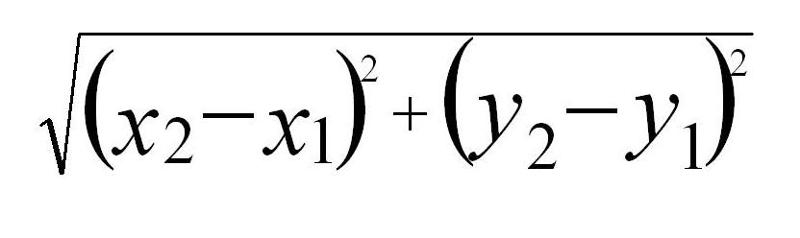10

Midpoint formula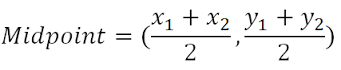11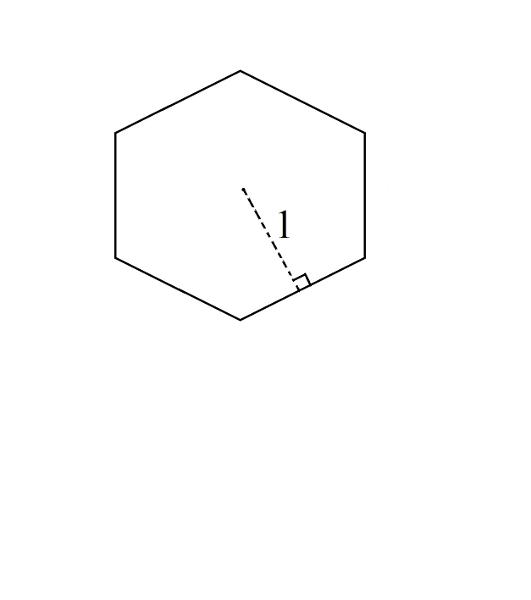Name the following polygon based on the number of sides:

hexagon

12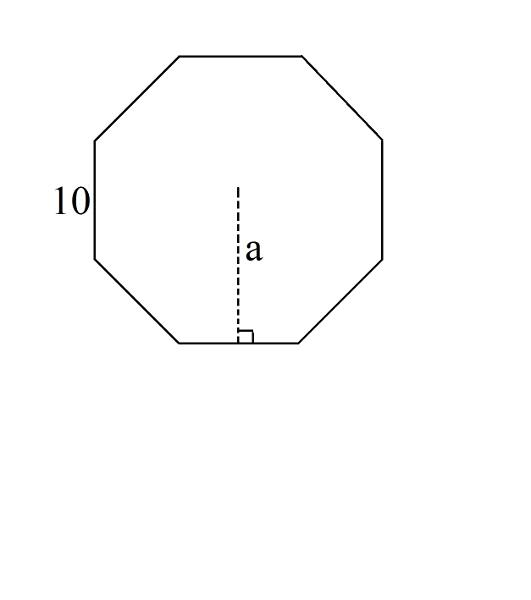Name the following polygon based on the number of sides:

Octagon

13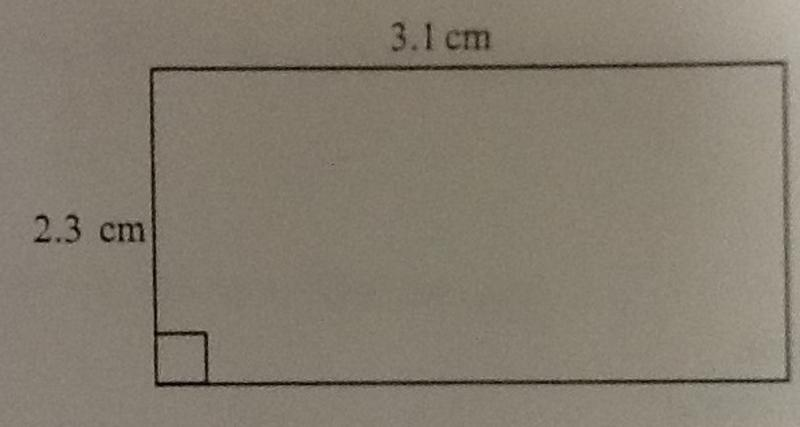Name the following polygon based on the number of sides:

Quadrilateral or, more specifically, a rectangle